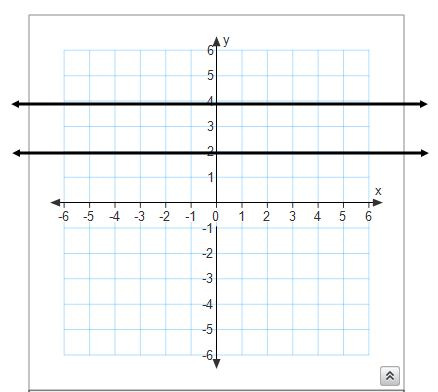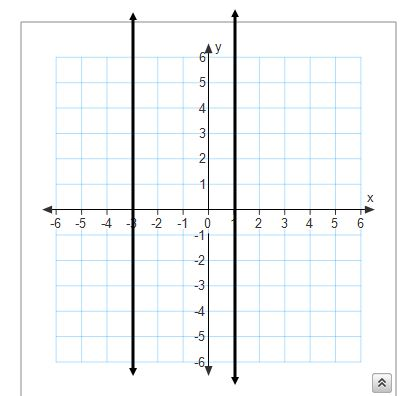Locus IV (Equidistant from two parallel lines)
Complete the form below to get homework credit
What is your last name and first name? *
What period are you in? *
The locus of points equidistant from two parallel lines will produce *
Complete the sentence
Which equation represents the locus of points equidistant from the lines y=3 and y=-1? *
If you forget a locus theorem you can sketch the locus by plotting one point that satisfies the condition and then successively plotting other points that satisfy the condition *
True or false
Look at the image below. What is the equation of the line that represents the locus of points equidistant from y=2 and y=4? *
Input your answer in the textbox. Do not use capital letters or spaces.